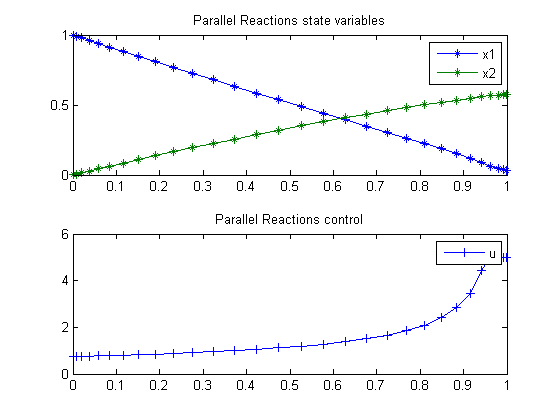80  Parallel Reactions in Tubular Reactor

Problem 4: DYNOPT User’s Guide version 4.1.0

Batch reactor with reactions: A -> B and A -> C.

M. Cizniar, M. Fikar, M. A. Latifi, MATLAB Dynamic Optimisation Code DYNOPT. User’s Guide, Technical Report, KIRP FCHPT STU Bratislava, Slovak Republic, 2006.

80.1  Problem description

Find T over t in [0; 1 ] to maximize

 J = x2(tf)

subject to:

 dx1 dt
= −(u+0.5*u2)*x1
 dx2 dt
= u*x1

where

 x(0) = [1  0]
 0 <= u <= 5

Reference: 

80.2  Problem setup

toms t
p = tomPhase('p', t, 0, 1, 30);
setPhase(p);

tomStates x1 x2
tomControls u

% Initial guess
x0 = {icollocate({x1 == 1; x2 == 0})
collocate(u == 5*t)};

% Box constraints
cbox = {0 <= collocate(u) <= 5};

% Boundary constraints
cbnd = initial({x1 == 1; x2 == 0});

% ODEs and path constraints
ceq = collocate({
dot(x1) == -(u+0.5*u.^2).*x1
dot(x2) == u.*x1});

% Objective
objective = -final(x2);

80.3  Solve the problem

options = struct;
options.name = 'Parallel Reactions';
solution = ezsolve(objective, {cbox, cbnd, ceq}, x0, options);
t  = subs(collocate(t),solution);
x1 = subs(collocate(x1),solution);
x2 = subs(collocate(x2),solution);
u  = subs(collocate(u),solution);
Problem type appears to be: lpcon
Starting numeric solver
===== * * * =================================================================== * * *
TOMLAB - Tomlab Optimization Inc. Development license  999001. Valid to 2011-02-05
=====================================================================================
Problem: ---  1: Parallel Reactions             f_k      -0.573540787113988930
sum(|constr|)      0.000000228705850230
f(x_k) + sum(|constr|)     -0.573540558408138670
f(x_0)      0.000000000000000000

Solver: snopt.  EXIT=0.  INFORM=1.
SNOPT 7.2-5 NLP code
Optimality conditions satisfied

FuncEv    1 ConstrEv   35 ConJacEv   35 Iter   34 MinorIter  118
CPU time: 0.125000 sec. Elapsed time: 0.125000 sec.

80.4  Plot result

subplot(2,1,1)
plot(t,x1,'*-',t,x2,'*-');
legend('x1','x2');
title('Parallel Reactions state variables');

subplot(2,1,2)
plot(t,u,'+-');
legend('u');
title('Parallel Reactions control');